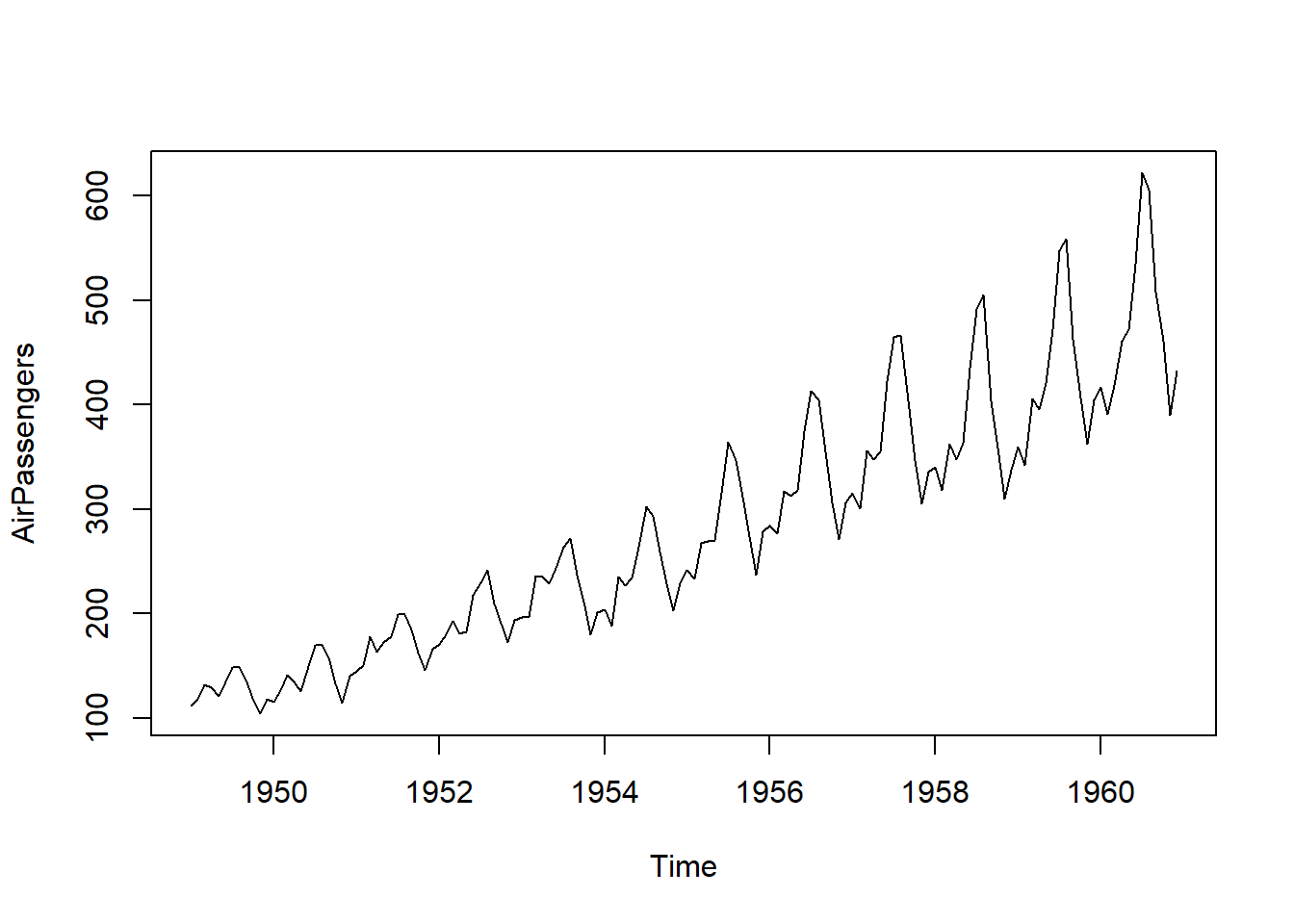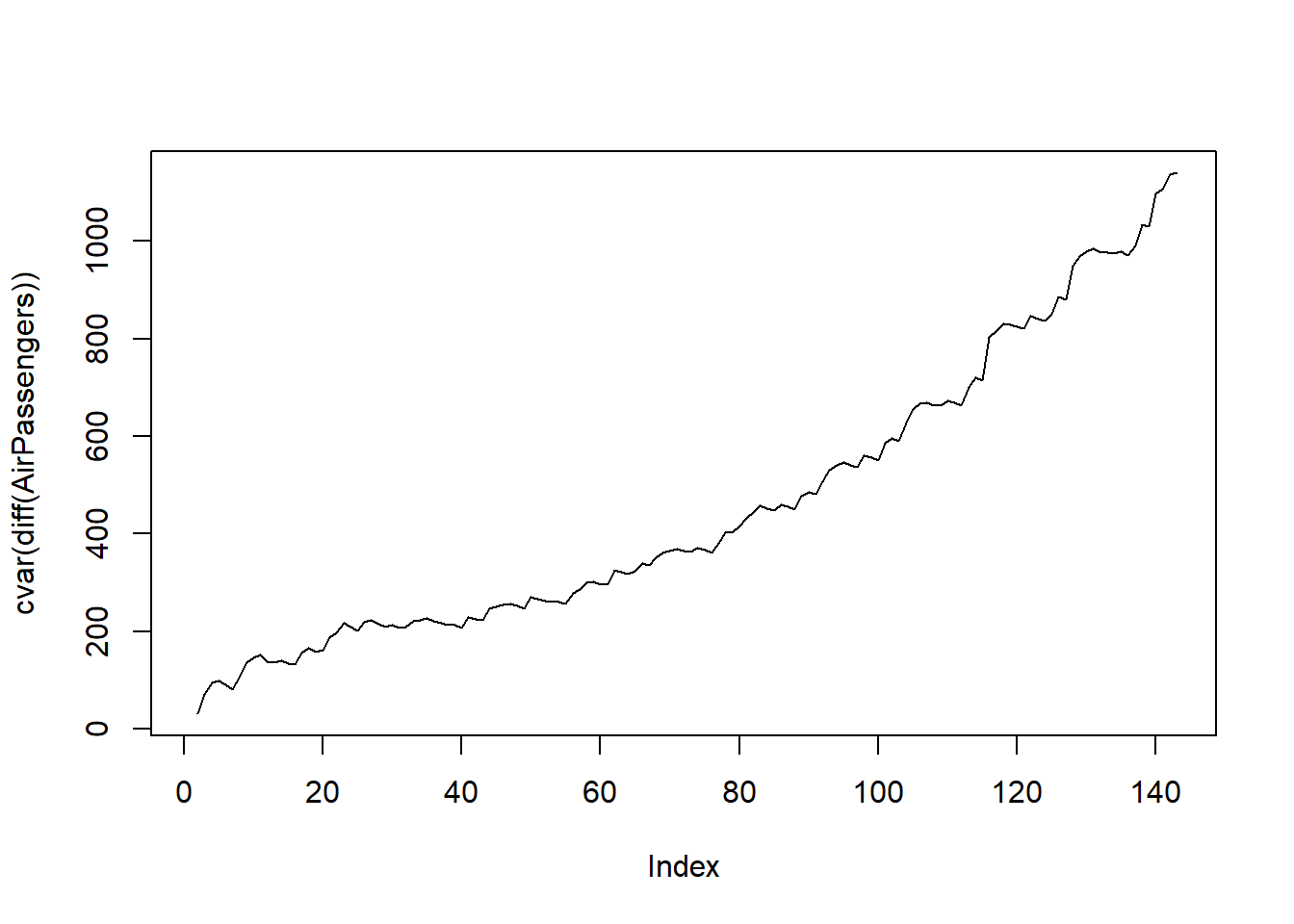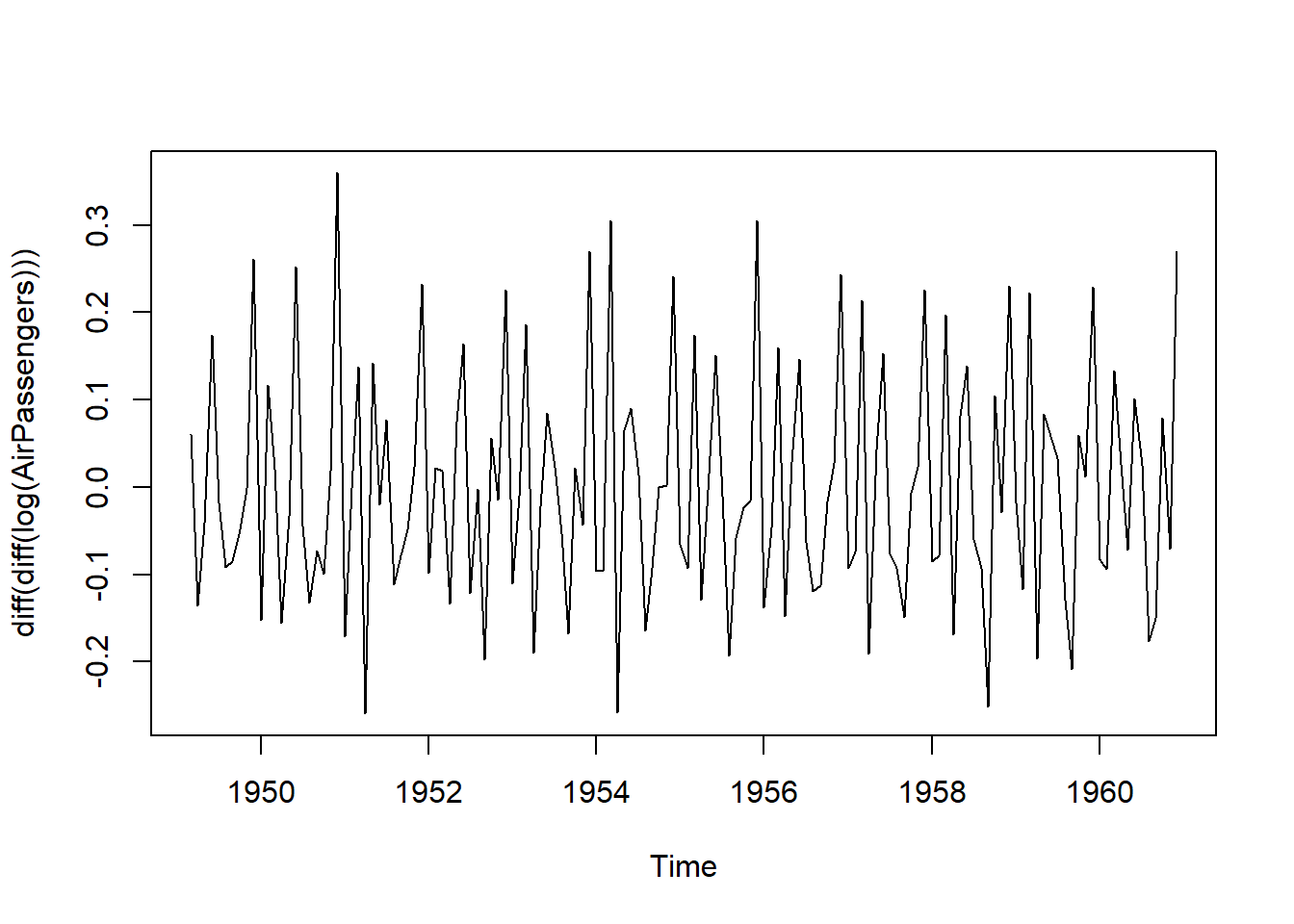Want to share your content on R-bloggers? click here if you have a blog, or here if you don't.

# Introduction

In the most basic sense for time series, a series is stationary if the properties of the generating process (the process that generates the data) do not change over time, the process remains constant. This does not mean the data does not change, it simply means the process does not change. You can bake a vanilla cake or a chocolate cake but you still cook it in the oven.

A non-stationary time series is like a toy car that doesn’t run in a straight line. Sometimes it goes fast and sometimes it goes slow, so it’s hard to predict what it will do next. But, just like how you can fix a toy car by adjusting it, we can fix a non-stationary time series by making it “stationary.”

One way we can do this is by taking the difference in the time series vector. This is like taking the toy car apart and looking at how each piece moves. By subtracting one piece from another, we can see if they are moving at the same speed or not. If they are not, we can adjust them so they are moving at the same speed. This makes it easier to predict what the toy car will do next because it’s moving at a steady pace.

Another way we can make a non-stationary time series stationary is by taking the second difference of the log of the data. This is like looking at the toy car from a different angle. By taking the log of the data, we can see how much each piece has changed over time. Then, by taking the second difference, we can see if the changes are happening at the same rate or not. If they are not, we can adjust them so they are happening at the same rate.

In simple terms, these methods help to stabilize the time series by making the data move at a consistent speed, which allows for better predictions.

In summary, a non-stationary time series is like a toy car that doesn’t run in a straight line. By taking the difference in the time series vector or taking the second difference of the log of the data, we can fix the toy car and make it run in a straight line. This is helpful for making accurate predictions.

# Function

We are going to use the `adf.test()` function from the `{aTSA}` library. Here is the function:

`adf.test(x, nlag = NULL, output = TRUE)`

Here are the arugments to the parameters.

• `x`– a numeric vector or time series.
• `alternative` – the lag order with default to calculate the test statistic. See details for the default.
• `output` – a logical value indicating to print the test results in R console. The default is TRUE.

# Examples

As an example, we are going to use the `R` built in data set AirPassengers as our timeseries. This data is both cyclical and trending so it is good for this purpose.

```library(aTSA)

plot(AirPassengers)```Now that we know what it looks like, lets see if it is stationary right off the bat.

`adf.test(AirPassengers)`
```Augmented Dickey-Fuller Test
alternative: stationary

Type 1: no drift no trend
[1,]   0  0.04712   0.657
[2,]   1 -0.35240   0.542
[3,]   2 -0.00582   0.641
[4,]   3  0.26034   0.718
[5,]   4  0.82238   0.879
Type 2: with drift no trend
[1,]   0 -1.748   0.427
[2,]   1 -2.345   0.194
[3,]   2 -1.811   0.402
[4,]   3 -1.536   0.509
[5,]   4 -0.986   0.701
Type 3: with drift and trend
[1,]   0 -4.64    0.01
[2,]   1 -7.65    0.01
[3,]   2 -7.09    0.01
[4,]   3 -6.94    0.01
[5,]   4 -5.95    0.01
----
Note: in fact, p.value = 0.01 means p.value <= 0.01 ```

So we can see that right off the bat that “Type 1” and “Type 2” fail as there is significant trend in this data as we can plainly see. Let’s see what happens when we take a simpmle `diff()` of the series.

`plot(diff(AirPassengers))`Looking like its still going to fail, but let’s run the test anyways.

`adf.test(diff(AirPassengers))`
```Augmented Dickey-Fuller Test
alternative: stationary

Type 1: no drift no trend
[1,]   0 -8.58    0.01
[2,]   1 -8.68    0.01
[3,]   2 -8.13    0.01
[4,]   3 -8.48    0.01
[5,]   4 -6.59    0.01
Type 2: with drift no trend
[1,]   0 -8.58    0.01
[2,]   1 -8.69    0.01
[3,]   2 -8.17    0.01
[4,]   3 -8.60    0.01
[5,]   4 -6.70    0.01
Type 3: with drift and trend
[1,]   0 -8.55    0.01
[2,]   1 -8.66    0.01
[3,]   2 -8.14    0.01
[4,]   3 -8.57    0.01
[5,]   4 -6.69    0.01
----
Note: in fact, p.value = 0.01 means p.value <= 0.01 ```

The adf.test comes back with a p.value <= 0.01 as the data is no longer presenting a trend, but as we can plainly see, the data has non constant variance overtime which we know we need. Here we will use the `{TidyDensity}` package to use the `cvar()` (cumulative variance) function to see the ongoing variance.

```library(TidyDensity)

plot(cvar(diff(AirPassengers)), type = "l")```Reject the null that the data is stationary. So lets proceed with a diff diff log of the data and see what we get. First let’s visualize.

`plot(diff(diff(log(AirPassengers))))``plot(cvar(diff(diff(log(AirPassengers)))), type = "l")`Looking good!

`adf.test(diff(diff(log(AirPassengers))))`
```Augmented Dickey-Fuller Test
alternative: stationary

Type 1: no drift no trend
[1,]   0 -15.90    0.01
[2,]   1 -12.78    0.01
[3,]   2  -9.28    0.01
[4,]   3 -10.76    0.01
[5,]   4  -9.72    0.01
Type 2: with drift no trend
[1,]   0 -15.85    0.01
[2,]   1 -12.73    0.01
[3,]   2  -9.24    0.01
[4,]   3 -10.73    0.01
[5,]   4  -9.68    0.01
Type 3: with drift and trend
[1,]   0 -15.79    0.01
[2,]   1 -12.68    0.01
[3,]   2  -9.21    0.01
[4,]   3 -10.68    0.01
[5,]   4  -9.64    0.01
----
Note: in fact, p.value = 0.01 means p.value <= 0.01 ```

Voila!